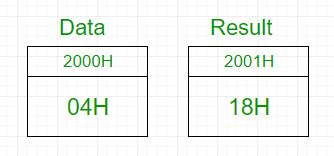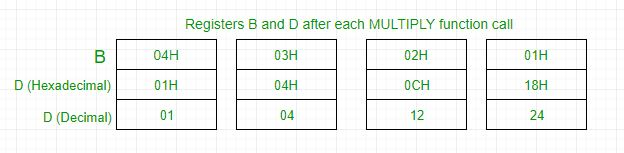# 8085 program to find the factorial of a number

Problem – Write an assembly language program for calculating the factorial of a number using 8085 microprocessor.

Example –

```Input : 04H
Output : 18H
as 04*03*02*01 = 24 in decimal => 18H
```In 8085 microprocessor, no direct instruction exists to multiply two numbers, so multiplication is done by repeated addition as 4×3 is equivalent to 4+4+4 (i.e., 3 times).
Load 04H in D register -> Add 04H 3 times -> D register now contains 0CH -> Add 0CH 2 times -> D register now contains 18H -> Add 18H 1 time -> D register now contains 18H -> Output is 18HAlgorithm –

1. Load the data into register B
2. To start multiplication set D to 01H
4. Decrements B to multiply previous number
6. Take memory pointer to next location and store result
7. Load E with contents of B and clear accumulator
8. Repeatedly add contents of D to accumulator E times
9. Store accumulator content to D
10. Go to step 4
2000H Data Data Byte
2001H Result Result of factorial
2002H LXI H, 2000H Load data from memory
2005H MOV B, M Load data to B register
2006H MVI D, 01H Set D register with 1
2008H FACTORIAL CALL MULTIPLY Subroutine call for multiplication
200BH DCR B Decrement B
200CH JNZ FACTORIAL Call factorial till B becomes 0
200FH INX H Increment memory
2010H MOV M, D Store result in memory
2011H HLT Halt
2100H MULTIPLY MOV E, B Transfer contents of B to C
2101H MVI A, 00H Clear accumulator to store result
2104H DCR E Decrement E
2108H MOV D, A Transfer contents of A to D
2109H RET Return from subroutine

Explanation –

1. First set register B with data.
2. Set register D with data by calling MULTIPLY subroutine one time.
3. Decrement B and add D to itself B times by calling MULTIPLY subroutine as 4*3 is equivalent to 4+4+4 (i.e., 3 times).
4. Repeat the above step till B reaches 0 and then exit the program.
5. The result is obtained in D register which is stored in memory

GeeksforGeeks has prepared a complete interview preparation course with premium videos, theory, practice problems, TA support and many more features. Please refer Placement 100 for details

My Personal Notes arrow_drop_upCheck out this Author's contributed articles.

If you like GeeksforGeeks and would like to contribute, you can also write an article using contribute.geeksforgeeks.org or mail your article to contribute@geeksforgeeks.org. See your article appearing on the GeeksforGeeks main page and help other Geeks.

Please Improve this article if you find anything incorrect by clicking on the "Improve Article" button below.

Improved By : aastha98